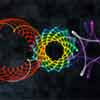## March 29, 2010

### A few spirographs in After EffectsSpirographs in After Effects had it's 15-minutes of fame several years ago and lost in the mists of time around here anyway, except for tutorial/project posts from Chris Zwar, Ayato, and Michele Yamazaki (now only in her book).

... this post was updated on ProVideo Coalition as Spirographs in After Effects.

Note: code from comments by Jason Lee Peacock,

I've recently been looking into this topic myself, but within a 3D space (thanks to the modified code of Dan Ebberts) http://twitpic.com/1ao7d0 , having seen this via Quba Michalski http://qubahq.com/2005/08/sine-sphere/

http://twitpic.com/1bscaz .. Literally clueless to the math ( http://tinyurl.com/yeg37lt ) I just kept tweaking Dan's code until I got the result I was after..

r = 200; //radius of sphere
Theta = Math.PI*time*5*Math.sin(10)/2;
Phi = Math.PI*time*1/2;
theta = ease(time,Theta,Theta);
phi = ease(time,Phi,Phi);
sinPhi = Math.sin(phi);
x = r*Math.cos(theta)*sinPhi;
y = r*Math.sin(theta)*sinPhi;
z = r*Math.cos(phi);
center = thisComp.layer("Null: Center").position;
center + [x,y,z]

Jlpeacock said...

I've recently been looking into this topic myself, but within a 3D space (thanks to the modified code of Dan Ebberts) http://twitpic.com/1ao7d0 , having seen this via Quba Michalski http://qubahq.com/2005/08/sine-sphere/

Rich said...

Plese tell more on the modified code of Dan Ebberts...

Jlpeacock said...

http://twitpic.com/1bscaz .. Literally clueless to the math ( http://tinyurl.com/yeg37lt ) I just kept tweaking Dan's code until I got the result I was after..

r = 200; //radius of sphere
Theta = Math.PI*time*5*Math.sin(10)/2;
Phi = Math.PI*time*1/2;
theta = ease(time,Theta,Theta);
phi = ease(time,Phi,Phi);
sinPhi = Math.sin(phi);
x = r*Math.cos(theta)*sinPhi;
y = r*Math.sin(theta)*sinPhi;
z = r*Math.cos(phi);
center = thisComp.layer("Null: Center").position;
center + [x,y,z]

Christopher Kirkman said...

Brilliant Jason! This is exactly what I was looking to add to my tut. I worked with Dan a little bit, but couldn't come up with a good way to do this in 3D space cuz I'm just as lost on the math as most.

Thanks!